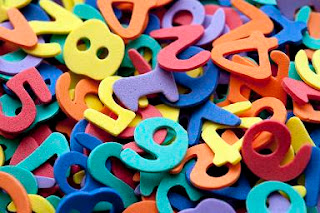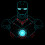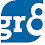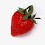# Shortcut for Square - A Simple Method

We have already discussed a method for finding the squares of the given number (check that method Here). Now we shall discuss another simplest method to calculate square of the given number. First of all one thing you should keep in mind, for you r convinience we've mentioned each and every step in detail. But You should do all these calculations MENTALLY. I mean without using pen or pencil. So practice well to do these problems orally. Now lets go to the topic, Finding the square of a number ending in 5 is very simple. If the last digit of the number is 5, then the last two digits of the square will be 25.What ever is the earlier part of the number multiply it with one more than itself and that will be the first part of the answer. (The second part of the answer will be 25 only).

352 = 1225  Here 3 x 4 = 12 so the answer is 1225
452   = 2025
552   = 3025
752   = 5625
952   = 9025
1252   = 15625
1752   = 30625
2352  = 55225
1952   = 38025
2452   = 60025

Well friends, now we know the squares of numbers 25, 30, 35, 40, 45, 50, 55 etc. If we want to find the squares of any other number, we find it using these squares which we already know.

For 26we will go from 252, for 32we go from 30 and so on.

One way is writing 262 = (25+1)2. But we need not even calculate (a+b)2 by adopting the following method.

26 = 252 + 26th odd number. i.e., 625 + 51 = 676
(a+b)  = a+ 2ab + b2

26  = (25 + 1 )2
= 25 + 2 x 25 x 1 + 12

= 625 + 50 + 1
= 625 + 51
= 676So, the answer is same. Now we shall look at an easiest method which will help you to calculate Squares Mentally.

1  = 1 = 1
= 4 = 1+3
3  = 9 = 1+ 3 + 5
4  = 16 = 1 + 3 + 5 + 7
5  = 25 = 1 + 3 + 5 + 7 + 9

I mean, to get n2, you should add the FIRST n odd numbers. If we want 172, it will be the sum of the FIRST 17 odd numbers.

nth odd number is equal to (2n - 1)

Suppose you want to find out 62 knowing what 52 is, we can move from 52 to 62.

6will be the sum of 1st 6 odd numbers. But the sum of the first 6 odd numbers can be written as " Sum of the first 5 odd numbers" + "Sixth odd number". Since we already know that the sum of the FIRST 5 odd numbers is 52 , i.e., 25, we need to add the sixth odd number, i mean (2x 6-1) = 11 to 25 to give us 62 = 36.

Similarly
31= 900 + 31st Odd number = 900 + 61 = 961
362 = 1225 + 36th odd number = 1225 + 71
= 1296 (remember that 352 = 1225)
41=  1600 + 81 = 1681
462 =  2025 + 91 = 2116
126= 15625 + 251 = 15876
196=  38025 + 391 = 38416
216=  46225 + 461 = 46656      Read More

Don't forget to LIKE our Facebook page here for daily Math Shortcut Tips

1.2.pretty good and easy one.. thanks for the contribution

3.thank you...:)

4.do any type of square

let's take (74)^2=5476
(7)^2=49
(4)^2=16
7*4*2=56 so write as 4 9 1 6
5 6
---- -------
5 4 7 6
another ex (109)^2=11881
first square of 10=100
then square of 9=81
10*9*2=180
1 0 0 8 1
1 8 0
----------
1 1 8 8 1

1.Thank you so much for the comment Prag Kamra

Good Day :)

2.Thanks Prag,

3.excellent Prag Kamra...

4.but in last step how are you adding the number plz elaborate ot

5.what a trick salute u sir G.ese hi hume gyan dete rahiye

6.but we cant find the square of 123,243 by this techniq please guide

7.But according to prag kamra we cant find the square of 123 like many number please guide

8.we cant find square of 123 by dat method pls explain

9.we can find d square of 123 by dat method..
look
123 => 12 & 3
now(12)^2 = 144 & 3^2 = 09
so, 14409
now 2*12*3=72
14409
+72

10.As i did it like
216 => 2 &16
2^2=4 & 16^2=256
so 4256
thn 2*2*16=64
4256
+64
4896

11.Hw will you calculate the last step like 10081 and 180. Kindly clarify that one.

5.it takes time so better for everyone to remember at least 50

6.Nice. . .

7.thank you

8.THANKU YAR .......THIS IS NICE TRICK..........

9.Its truely useful for all i will surely share this site with my friends

10.impressive.. (y)

11.Simply superb

12.simply superb..

13.cool... thx sir...

14.method of prag is also nice one. thanks prag.
n mam i have a doubt in last method. if we need sq of 37 then, acording to (35)^2 + 37th term the answer is not comming correct..

15.look now a combination of mam n prag method.
what if given term is large like 329 or 412.
we dnt knw sq of 32 or 41. but we know d short method.
32^2= 04
12
9
adding in this manner what we get. 1024
what is the sq of 9. its 81.
so numbr comes as told by prag 102481.
now what we have to ad 2*32*9. cant we do this?
576? right.
102481
576
108241.
yo done it.. chk it now.. n enjoy.. all the sq of 3digit numbers u have now before a minute. jst practice.

16.look now a combination of mam n prag method.
what if given term is large like 329 or 412.
we dnt knw sq of 32 or 41. but we know d short method.
32^2= 04
12
9
adding in this manner what we get. 1024
what is the sq of 9. its 81.
so numbr comes as told by prag 102481.
now what we have to ad 2*32*9. cant we do this?
576? right.
102481
576
108241.
yo done it.. chk it now.. n enjoy.. all the sq of 3digit numbers u have now before a minute. jst practice.